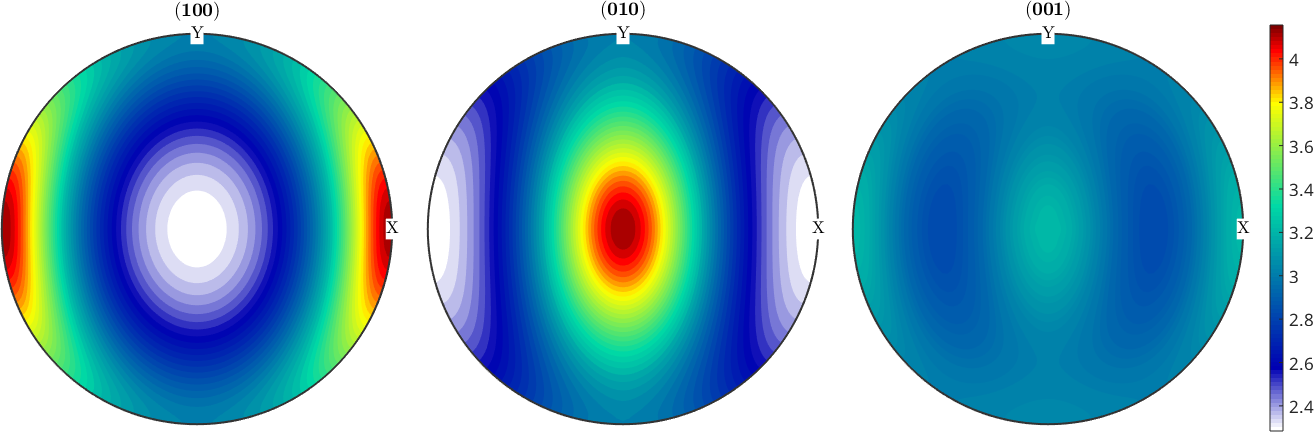Sigle Slip Model edit page

Details to this model can be found in

## The Continuity Equation

The evolution of the orientation distribution function (ODF) $$f(g)$$ with respect to a crystallopgraphic spin $$\Omega(g)$$ is governed by the continuity equation

$\frac{\partial}{\partial t} f + \nabla f \cdot \Omega + f \text{ div } \Omega = 0$

The solution of this equation depends on the initial texture $$f_0(g)$$ at time zero and the crystallographic spin $$\Omega(g)$$. In this model we assume the initial texture to be isotrope, i.e., $$f_0 = 1$$ and the crystallopgraphic spin be associated with a single slip system. The full ODF will be later modelled as a superposition of the single slip models.

In this example we consider Olivine with has orthorhombic symmetry

and the basic slip systems in olivine and orthopyroxene

To each of the slip systems we can associate an orientation dependent Schmid or deformation tensor $$S(g)$$

and make for the orientation dependent strain rate tensor $$e(g)$$ the ansatz $$e_{ij}(g) = \gamma(g) S_{ij}(g)$$. Fitting this ansatz to a given a macroscopic strain tensor

via minimizing the square difference

$\int_{SO(3)} \sum_{i,j} (e_{i,j}(g) - E_{i,j})^2 dg \to \text{min}$

the orientation dependent strain rate tensor is identified as

$e(g) = 2 \left< S(g), E \right> S(g)$

and the corresponding crystallographic spin tensor as

$\Omega_i(g) = \epsilon_{ijk} e_{jk}(g)$

This can be modeled in MTEX via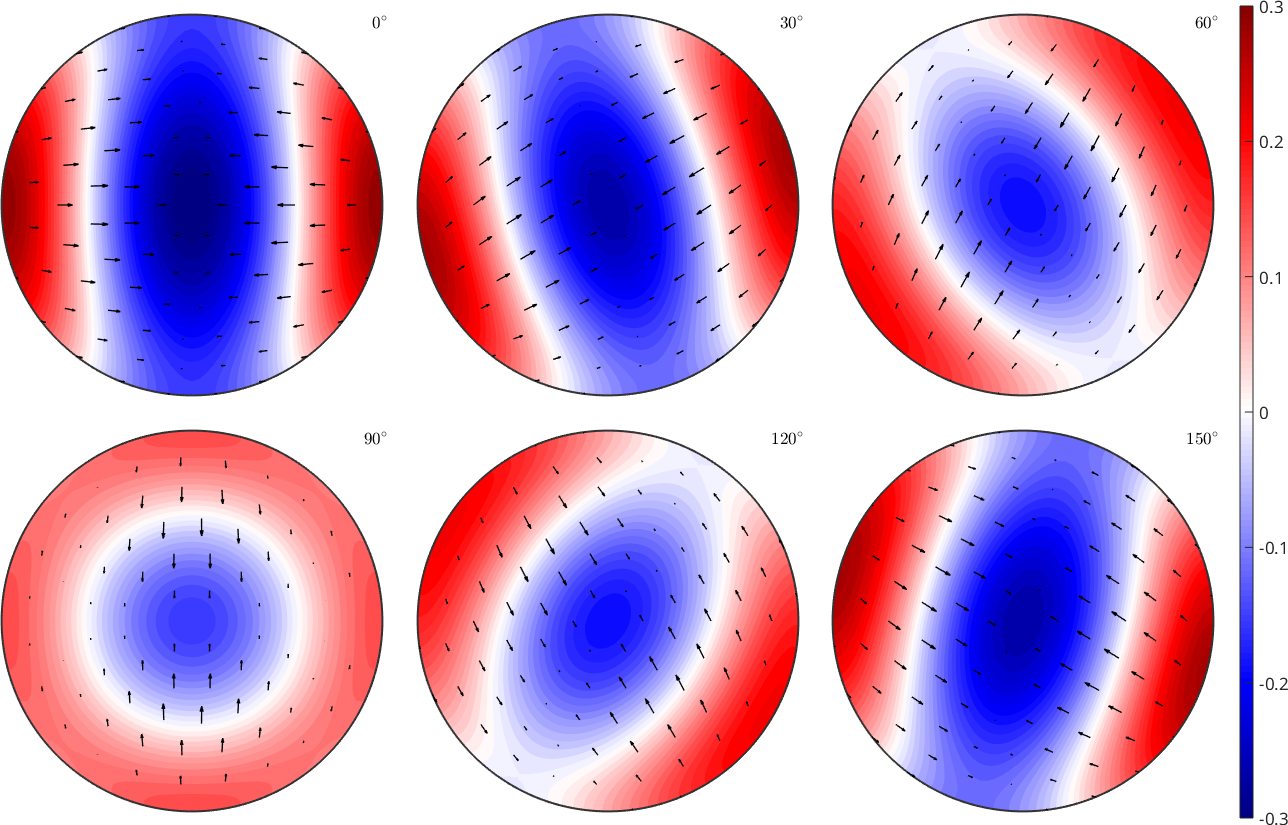The divergence plots can be read as follows. Negative (blue) regions indicate orientations that increase in volume, whereas positive regions indicate orientations that decrease in volume. Accordingly, we expect the texture to become more and more concentrated within the blue regions. In the example example illustrated above with only the second slip system beeing active, we would expect the c-axis to align more and more with the the z-direction.

## Solutions of the Continuity Equation

The solutions of the continuity equation can be analytically computed and are available via the command SO3FunSBF. This command takes as input the specific slips system sS and the makroscopic strain tensor E

Lets check our expectation from the last paragraph by visualizing the odf corresponding to the second slip system in sigma sections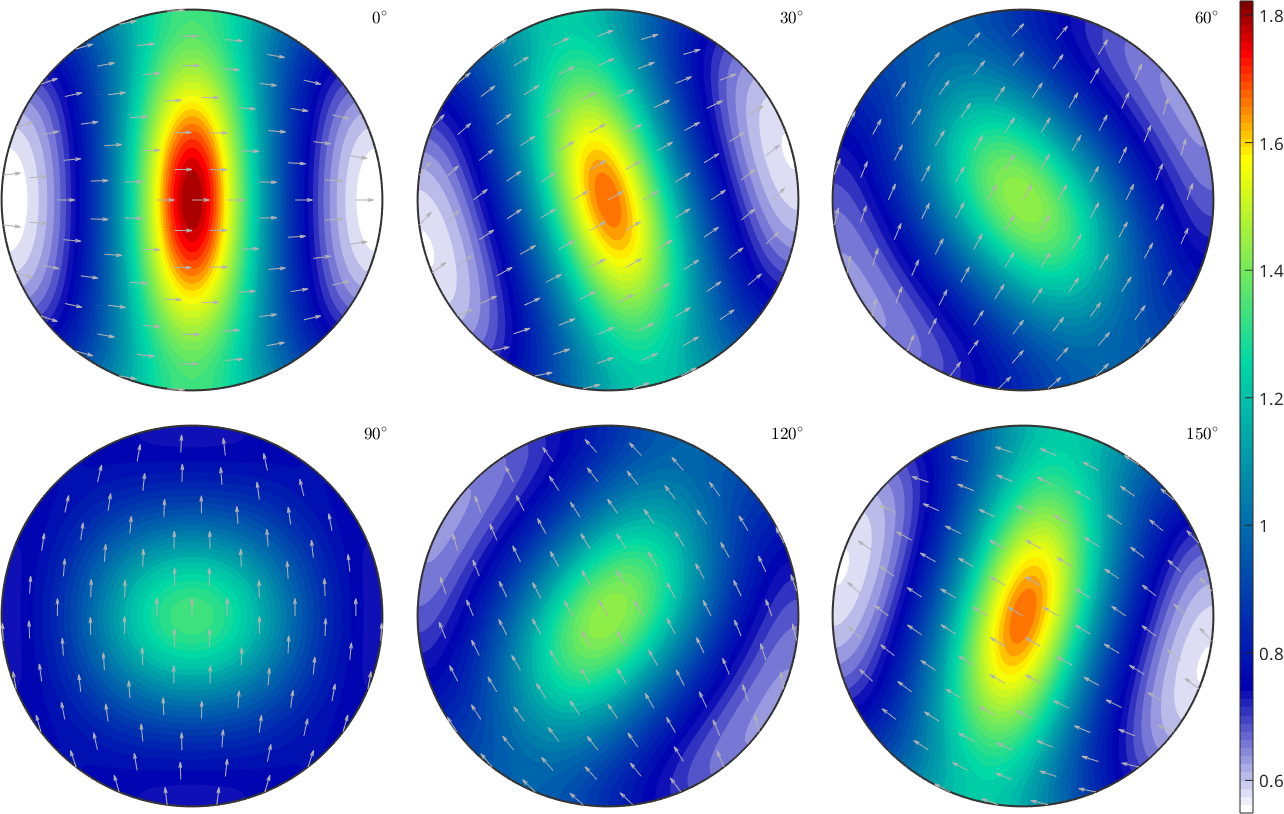We observe exactly the concentration of the c-axis around z as predicted by the model. This can be seen even more clear when looking a the pole figures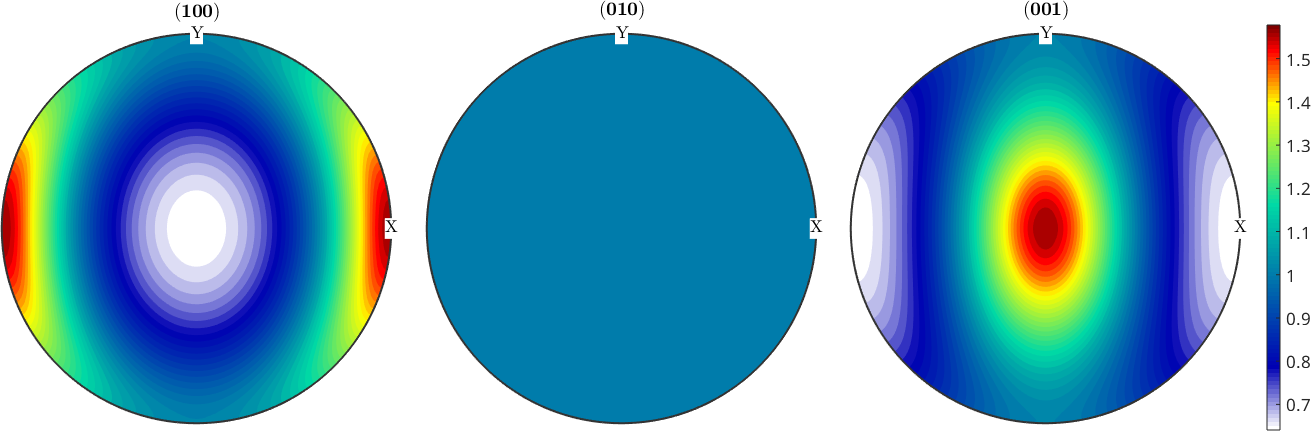For completeness the pole figures of the other two basis functions.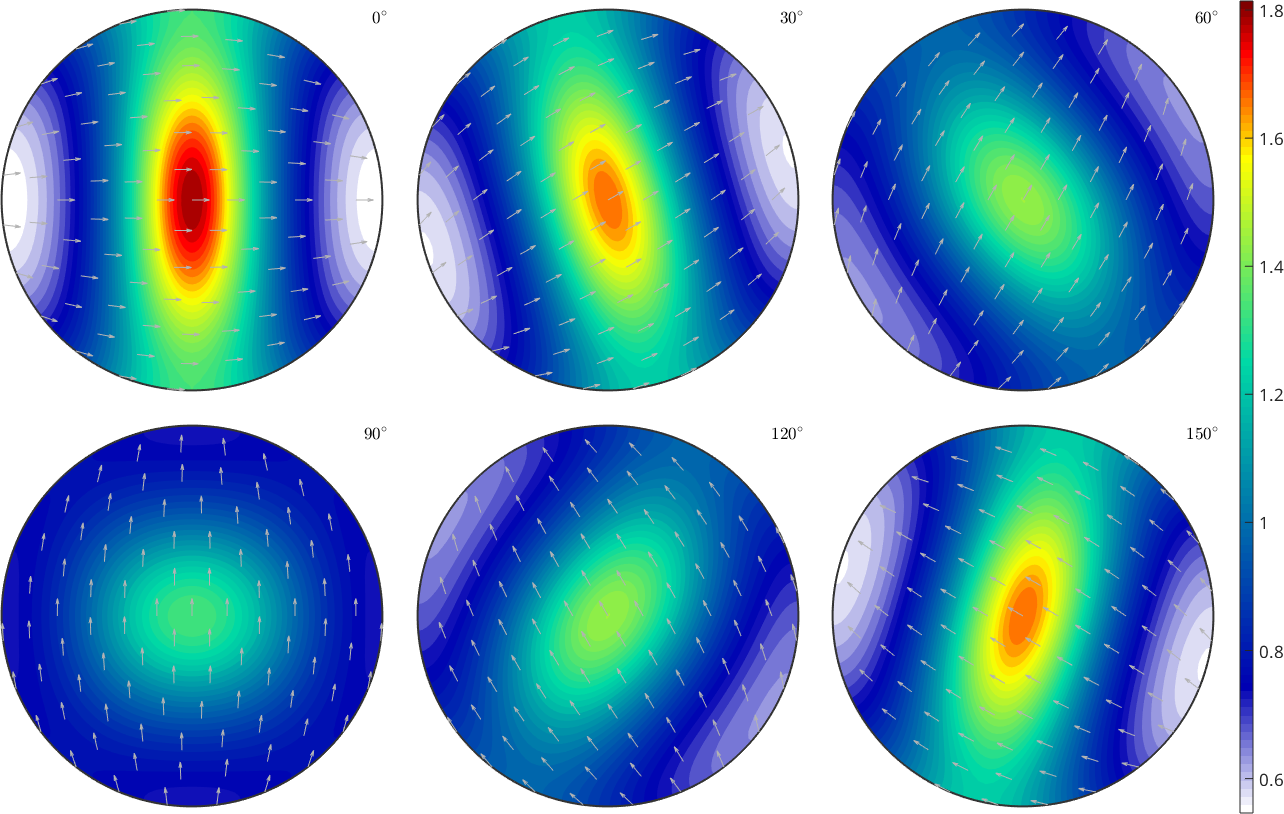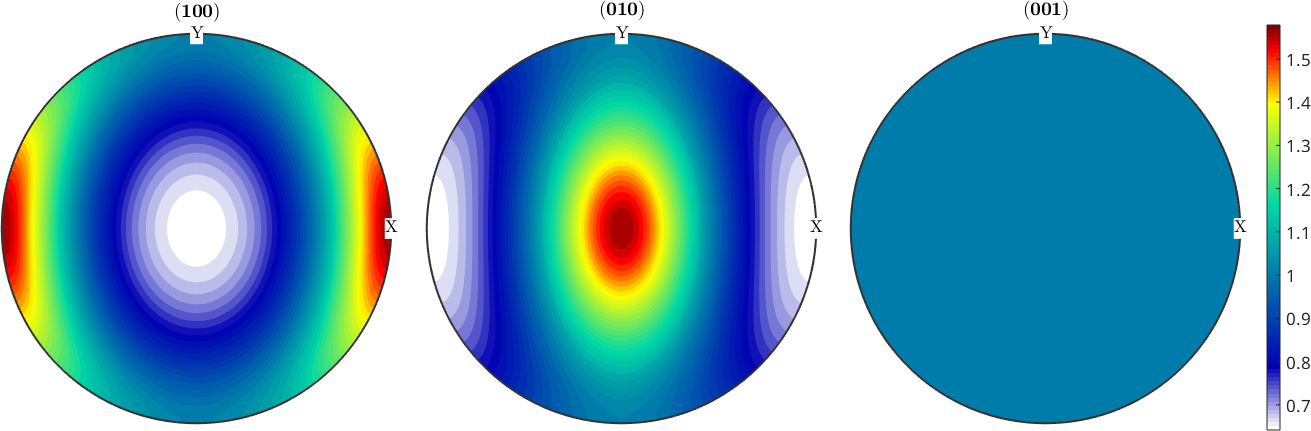We observe that the pole figure with respect to $$n \times b$$ is always uniform, where $$n$$ is the slip normal and $$b$$ is the slip direction.

Since in practice all three slip systems are active we can model the resulting ODF as a linear combination of the different basis functions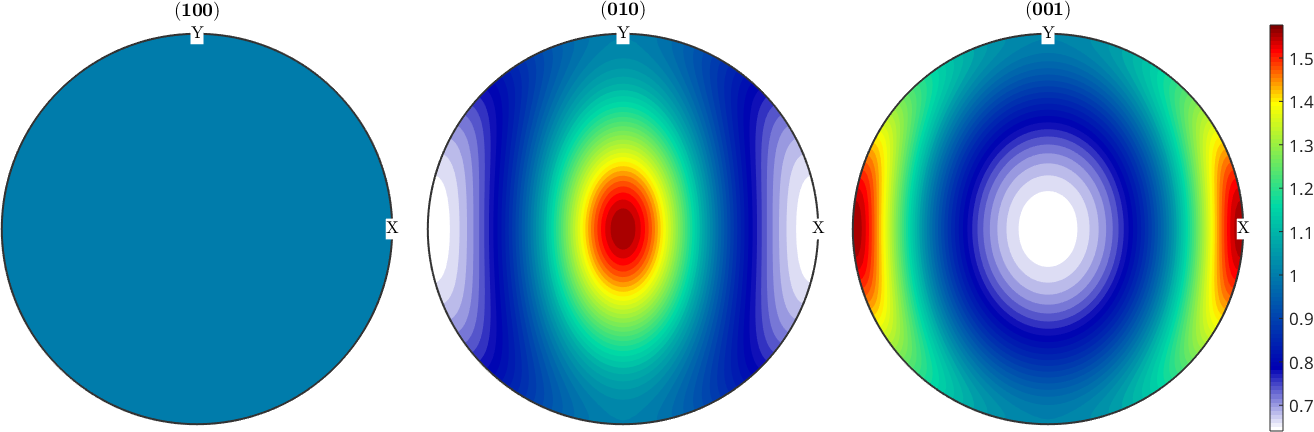## Checking the for steady state

We may also check for which orientations an ODF is already in a steady state of the continous equation, i.e., the time derivative $$\text{div}(f \Omega) = 0$$ is zero.